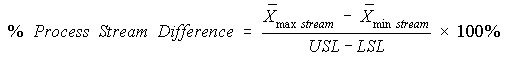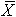# Process Stream Difference

A useful diagnostic measure to understand the magnitude of process stream differences is the % process stream difference. This measure is simply the range of the process stream means divided by the specification range expressed as a percentage. This is calculated as follows.where

• USL is the Upper Specification Limit,
• LSL is the Lower Specification Limit,
•max stream is the largest process stream mean, and
•min stream is the smallest process stream mean.